# Adding and Subtracting Multi-Digit Whole Numbers

 Listen to this Lesson:

Children learn how to add and subtract multi-digit whole numbers in fourth grade. By the time they start fifth grade, they should have a decent understanding of multi-digit whole number addition and subtraction based on the use of the standard algorithm for these operations.

## Tips to Teach Addition and Subtraction of Multi-Digit Numbers### Basic Definitions and Terms

You can start by reminding your students that adding multi-digit numbers means joining two or more numbers that are made up of more than one digit (like 12; 549; and 60,378) into one larger number. Terminology is important, so make sure they know that the numbers they’re adding are called addends, while the answer is their sum.

Similarly, explain to your students that subtracting multi-digit numbers means taking away one number that contains several digits (the subtrahend) from another multiple digit number (the minuend). The former needs to be smaller than the latter. They should also know that the outcome of this operation is called the rest or the difference.

To help them memorize these terms more easily and quickly, you can write two examples on the whiteboard using a different color for each part of the mathematical problem. Here’s how: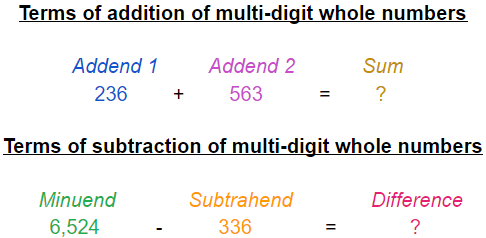After that, you can highlight that when adding numbers, the order of the problem doesn’t matter, but it does with subtraction. In other words, changing the order of the addends won’t change the sum (9+7=16 and 7+9=16), but switching a subtraction problem around won’t result in the same difference.

### Place Value Knowledge Review

To be able to add and subtract multi-digit whole numbers using the standard algorithm (which is the most commonly used method for addition and subtraction), your students must fully understand place value. Although they’ve already been introduced to this concept, we strongly suggest testing their knowledge with a few simple games.

For instance, you can print out copies of a place value chart showing millions down to ones, pick several multiple digit whole numbers, and have the children identify the positional value of each digit by putting the numbers in the chart. Another great option is to just write down a few multi-digit whole numbers and assign your students to draw a red circle around the ones, a blue square around the tens, an orange heart around the hundreds, and so on.

Our last suggestion is to divide the kids into groups and make each team represent a place from the place value chart. For example, team purple can represent ones, team yellow can represent tens, team green can represent hundreds, etc. Then, instruct your students to stand up every time you read a number that contains a digit the positional value of which they represent. So, if you pick 539, three groups of students will need to stand up.

### Standard Algorithm for Addition and Subtraction

Before you dive into the specifics of the standard algorithm, point out that it’s the most commonly used method for solving these types of operations. After that, walk your fifth graders through the process step by step.

Step 1: Remind your students that the numbers they’re adding or subtracting need to be lined up vertically with the place values matching. In other words, the ones of the second addend (or the subtrahend if solving a subtraction problem) need to be right below the ones of the first addend (or the minuend if dealing with subtraction). To avoid making mistakes, advise the kids to always begin with the ones place.

Step 2: Now it’s time to instruct your students to add or subtract the digits with the same place value, always starting from the right to the left place value, i.e. from the ones, to the tens, to the hundreds, etc.

It’s smart to start with simple addition and subtraction problems, practice a little, and then introduce your students to carrying over and borrowing.

#### Carrying Over

Explain to the children in your class that sometimes the sum of the digits that share the same place value can be greater than 10. This is when they should use the ‘carrying over’ concept or rule. To help them understand more quickly, use an example such as this: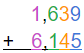Explain to your students that since the sum of 9 and 5 is 14, they need to regroup the ones. 14 can be broken apart into 1 tens and 4 ones, which is why they should write 4 in the ones place and carry over the 1 tens above the tens place.Now, point out that when they’ll add the tens, they need to take into account the 1 tens they carried over, too.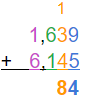The hundreds and thousands can be added the usual way since their sums aren’t greater than 10.#### Borrowing

Start by emphasizing that the concept of ‘borrowing’ is used only when a digit in the minuend is less than the digit with the same place value in the subtrahend. You can use the following example: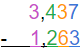Explain to your students that they need to apply the borrowing rule to subtract the tens in this problem. Since 3 tens (in the minuend) are less than 6 tens (in the subtrahend), the 3 needs to borrow 1 from the next digit placed on the left because it’s bigger. In this case, that’s 4. Remind the children that 4 is in the hundreds place, which means the 1 they’ll borrow from it represents a 100 or 10 tens. So, 3 tens plus 10 tens from the nice neighbor on the left equals 13 tens. Now they can subtract 6 tens from 13 tens.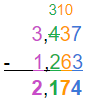Make sure to point out that the lending digit needs to be reduced by 1 like shown above.

Don’t forget to explain to your fifth graders that when the neighbor on the left they want to borrow from is 0, they can borrow from the next two digits. If there are two zeros on the left, they should borrow from the next three digits and so on.

## Practice Adding and Subtracting Multi-Digit Whole NumbersTo help your students become fluent in adding and subtracting multi-digit numbers using the standard algorithm, you can get them to solve engaging word problems and/or have them play a fun game. Children love playing games with animals (not actual ones, of course), so you might want to introduce them to the Tandem Cat Cycling Race. Here’s what you need to do:

1. Divide your students into groups of two kittens
2. Let them pick a team name
3. Explain to them that the cat cycling race is 10 miles and they can win it by solving 10 addition or subtraction problems correctly (they gain strength for 1 mile with each correct answer)
4. Warn them that each wrong answer will cause the kittens to fall from the bicycle and set the team back by 1 mile
5. Let them know that they’ll need to say the answers out loud once they solve the problems
6. Point out that once one of the teams solves a problem successfully, the other groups can’t answer it (they must continue to the next one)
7. Inform them that the game will last 2 minutes (you can change the game duration if necessary)

To make sure everything goes smoothly, create 30-40 addition and subtraction problems involving 4-, 5-, 6-, and 7-digit numbers and print a copy for each team. Have the correct answers at hand so you can check your students’ sums and differences quickly.